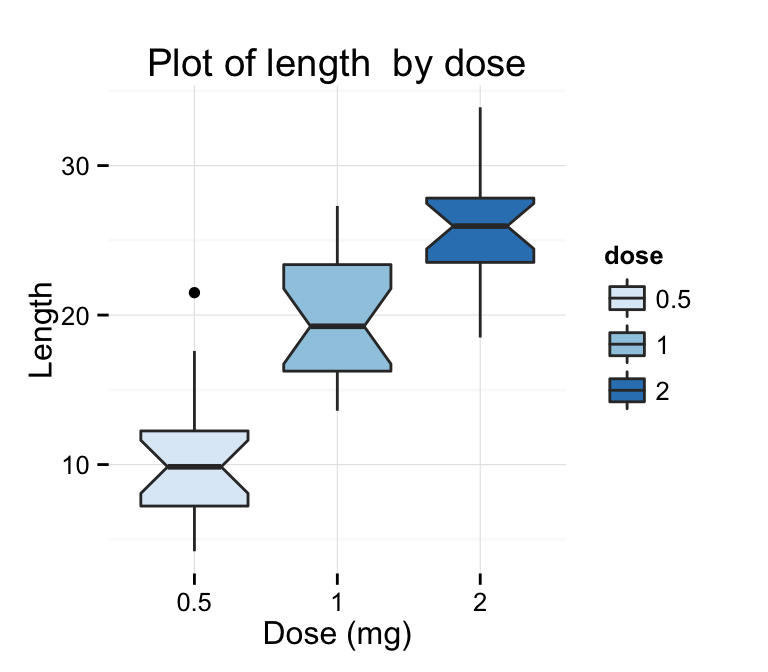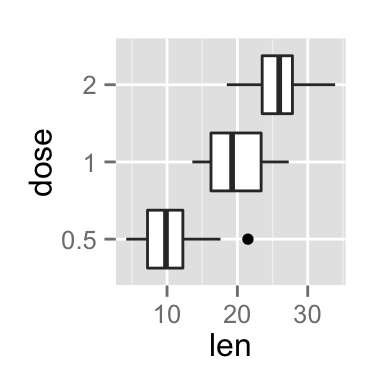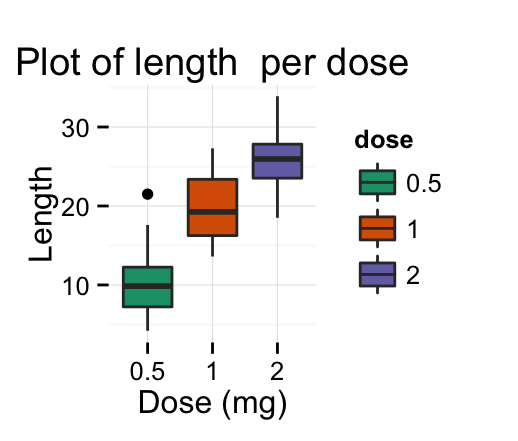# ggplot2 box plot : Quick start guide - R software and data visualization

This R tutorial describes how to create a box plot using R software and ggplot2 package.

The function geom_boxplot() is used. A simplified format is :

``````geom_boxplot(outlier.colour="black", outlier.shape=16,
outlier.size=2, notch=FALSE)``````
• outlier.colour, outlier.shape, outlier.size : The color, the shape and the size for outlying points
• notch : logical value. If TRUE, make a notched box plot. The notch displays a confidence interval around the median which is normally based on the median +/- 1.58*IQR/sqrt(n). Notches are used to compare groups; if the notches of two boxes do not overlap, this is a strong evidence that the medians differ.# Prepare the data

ToothGrowth data sets are used :

``````# Convert the variable dose from a numeric to a factor variable
ToothGrowth\$dose <- as.factor(ToothGrowth\$dose)
``````##    len supp dose
## 1  4.2   VC  0.5
## 2 11.5   VC  0.5
## 3  7.3   VC  0.5
## 4  5.8   VC  0.5
## 5  6.4   VC  0.5
## 6 10.0   VC  0.5``````

Make sure that the variable dose is converted as a factor variable using the above R script.

# Basic box plots

``````library(ggplot2)
# Basic box plot
p <- ggplot(ToothGrowth, aes(x=dose, y=len)) +
geom_boxplot()
p
# Rotate the box plot
p + coord_flip()
# Notched box plot
ggplot(ToothGrowth, aes(x=dose, y=len)) +
geom_boxplot(notch=TRUE)
# Change outlier, color, shape and size
ggplot(ToothGrowth, aes(x=dose, y=len)) +
geom_boxplot(outlier.colour="red", outlier.shape=8,
outlier.size=4)``````The function stat_summary() can be used to add mean points to a box plot :

``````# Box plot with mean points
p + stat_summary(fun.y=mean, geom="point", shape=23, size=4)``````Choose which items to display :

``p + scale_x_discrete(limits=c("0.5", "2"))``# Box plot with dots

Dots (or points) can be added to a box plot using the functions geom_dotplot() or geom_jitter() :

``````# Box plot with dot plot
p + geom_dotplot(binaxis='y', stackdir='center', dotsize=1)
# Box plot with jittered points
# 0.2 : degree of jitter in x direction
p + geom_jitter(shape=16, position=position_jitter(0.2))``````# Change box plot colors by groups

## Change box plot line colors

Box plot line colors can be automatically controlled by the levels of the variable dose :

``````# Change box plot line colors by groups
p<-ggplot(ToothGrowth, aes(x=dose, y=len, color=dose)) +
geom_boxplot()
p``````It is also possible to change manually box plot line colors using the functions :

• scale_color_manual() : to use custom colors
• scale_color_brewer() : to use color palettes from RColorBrewer package
• scale_color_grey() : to use grey color palettes
``````# Use custom color palettes
p+scale_color_manual(values=c("#999999", "#E69F00", "#56B4E9"))
# Use brewer color palettes
p+scale_color_brewer(palette="Dark2")
# Use grey scale
p + scale_color_grey() + theme_classic()``````Read more on ggplot2 colors here : ggplot2 colors

## Change box plot fill colors

In the R code below, box plot fill colors are automatically controlled by the levels of dose :

``````# Use single color
ggplot(ToothGrowth, aes(x=dose, y=len)) +
geom_boxplot(fill='#A4A4A4', color="black")+
theme_classic()
# Change box plot colors by groups
p<-ggplot(ToothGrowth, aes(x=dose, y=len, fill=dose)) +
geom_boxplot()
p``````It is also possible to change manually box plot fill colors using the functions :

• scale_fill_manual() : to use custom colors
• scale_fill_brewer() : to use color palettes from RColorBrewer package
• scale_fill_grey() : to use grey color palettes
``````# Use custom color palettes
p+scale_fill_manual(values=c("#999999", "#E69F00", "#56B4E9"))
# use brewer color palettes
p+scale_fill_brewer(palette="Dark2")
# Use grey scale
p + scale_fill_grey() + theme_classic()``````Read more on ggplot2 colors here : ggplot2 colors

# Change the legend position

``````p + theme(legend.position="top")
p + theme(legend.position="bottom")
p + theme(legend.position="none") # Remove legend``````The allowed values for the arguments legend.position are : “left”,“top”, “right”, “bottom”.

Read more on ggplot legend : ggplot2 legend

# Change the order of items in the legend

The function scale_x_discrete can be used to change the order of items to “2”, “0.5”, “1” :

``p + scale_x_discrete(limits=c("2", "0.5", "1"))``# Box plot with multiple groups

``````# Change box plot colors by groups
ggplot(ToothGrowth, aes(x=dose, y=len, fill=supp)) +
geom_boxplot()
# Change the position
p<-ggplot(ToothGrowth, aes(x=dose, y=len, fill=supp)) +
geom_boxplot(position=position_dodge(1))
p``````Change box plot colors and add dots :

``````# Add dots
p + geom_dotplot(binaxis='y', stackdir='center',
position=position_dodge(1))
# Change colors
p+scale_fill_manual(values=c("#999999", "#E69F00", "#56B4E9"))``````# Customized box plots

``````# Basic box plot
ggplot(ToothGrowth, aes(x=dose, y=len)) +
geom_boxplot(fill="gray")+
labs(title="Plot of length per dose",x="Dose (mg)", y = "Length")+
theme_classic()
# Change  automatically color by groups
bp <- ggplot(ToothGrowth, aes(x=dose, y=len, fill=dose)) +
geom_boxplot()+
labs(title="Plot of length  per dose",x="Dose (mg)", y = "Length")
bp + theme_classic()``````Change fill colors manually :

``````# Continuous colors
bp + scale_fill_brewer(palette="Blues") + theme_classic()
# Discrete colors
bp + scale_fill_brewer(palette="Dark2") + theme_minimal()
bp + scale_fill_brewer(palette="RdBu") + theme_minimal()``````Read more on ggplot2 colors here : ggplot2 colors

# Infos

This analysis has been performed using R software (ver. 3.1.2) and ggplot2 (ver. 1.0.0)